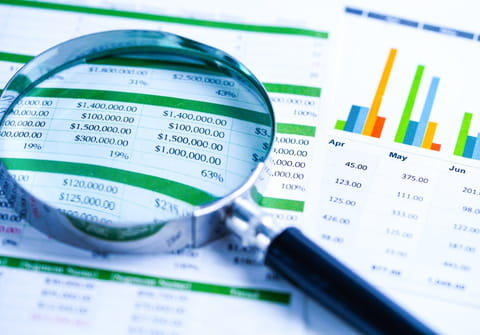# How to update dates in Excel spreadsheet: formulaDo you use Excel spreadsheets to keep track of your weekly, monthly or annual expenses but find yourself updating all relevant date and content information manually? There is an easier, more effective solution and in this article we will provide you with a macro formula which will help with the automatic update of information.

## What is the macro to update dates in Excel spreadsheet?

The macro will bring up an input box. there type the year for e.g. 2020 or 2021. You can format date column A as you like.

Test the macro and decide.

```Sub test()
Dim y As Integer, d As Integer
y = InputBox("type the year, e.g. 2010")
If y Mod 4 = 0 Then
d = 366
Else
d = 365
End If
Range("a1") = "1/1/" & y
Range(Range("A1"), Cells(d, "A")).DataSeries Rowcol:=xlColumns, _
Type:=xlChronological, Date:= _
xlDay, Step:=1, Trend:=False
Range("B1").Formula = "=weekday(A1)"
Range("B1").AutoFill Range(Range("B1"), Cells(d, "B"))
End Sub```

Should this macro not provide the solution to your problem, this article contains multiple macro solutions which might be of help.

Do you need more help with excel? Check out our forum!"
Subject Replies
Around the same subject